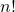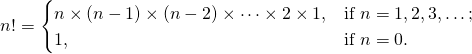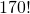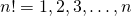# The many ways of writing the factorial function, n!

#### Introduction

The factorial of n,, is given byIn Fortran, there is no intrinsic function for factorial, so we must write our own.

#### Recursive Function

The factorial is one of the first examples that we encounter when we are learning about recursive functions.

    RECURSIVE FUNCTION factorial(n) RESULT(fact)
! Returns the value n! as an integer
INTEGER :: n, fact
IF (n == 0) THEN
fact = 1
ELSE
fact = n * factorial(n-1)
END IF
END FUNCTION factorial

This works well up until factorial(26). Then we get a signed-error. factorial(26) = -1569523520172457984.

By switching the declaration of n and fact from integer to real(kind=8), we can express the factorial all the way to.

    RECURSIVE FUNCTION factorial(n) RESULT(fact)
! Returns the value n! as an integer
REAL(KIND=8) :: n, fact
IF (n == 0) THEN
fact = 1
ELSE
fact = n * factorial(n-1)
END IF
END FUNCTION factorial

This works all the way up to factorial(170) = 7.257415615307994E+306.

Note that you can get higher factorials by using REAL(KIND=16).

#### Iterative Function

We can also write this iteratively.

    FUNCTION factorial(n)
! Returns the value n! as an integer
INTEGER(KIND=8) :: n, i
REAL(KIND=8) :: factorial

factorial = 1
DO i = 1, n
factorial = factorial * i
END DO

END FUNCTION factorial

#### Vectorized Function

This is a nice short way of writing the iterative function above, but without having to write a DO loop.

   FUNCTION factorial(n)
! Returns the value n! as an integer
INTEGER(KIND=8) :: n
REAL(KIND=8) :: factorial

factorial = product( [1:n] )

END FUNCTION factorial

Fast Factorial Functions: There are five algorithms which everyone who wants to compute the factorialshould know.